# 为什么你学不会递归？告别递归，谈谈我的经验

### 递归的三大要素

// 算 n 的阶乘(假设n不为0)
int f(int n){

}


// 算 n 的阶乘(假设n不为0)
int f(int n){
if(n == 1){
return 1;
}
}


// 算 n 的阶乘(假设n>=2)
int f(int n){
if(n == 2){
return 2;
}
}


// 算 n 的阶乘(假设n不为0)
int f(int n){
if(n <= 2){
return n;
}
}


f(n) = n * f(n-1)。

// 算 n 的阶乘(假设n不为0)
int f(int n){
if(n <= 2){
return n;
}
// 把 f(n) 的等价操作写进去
return f(n-1) * n;
}


### 案例1：斐波那契数列

1、第一递归函数功能

int f(int n){

}


2、找出递归结束的条件

int f(int n){
if(n <= 2){
return 1;
}
}


int f(int n){
// 1.先写递归结束条件
if(n <= 2){
return 1;
}
// 2.接着写等价关系式
return f(n-1) + f(n - 2);
}


### 案例2：小青蛙跳台阶

1、第一递归函数功能

int f(int n){

}


2、找出递归结束的条件

int f(int n){
if(n == 1){
return 1;
}
}


int f(int n){
if(n == 1){
return 1;
}
ruturn f(n-1) + f(n-2);
}


int f(int n){
//f(0) = 0,f(1) = 1，f(2) = 2等价于 n<=2时，f(n) = n。
if(n <= 2){
return n;
}
ruturn f(n-1) + f(n-2);
}


### 案例3：反转单链表。

class Node{
int date;
Node next;
}


1、定义递归函数功能

Node reverseList(Node head){

}


2. 寻找结束条件

Node reverseList(Node head){
}
}


3. 寻找等价关系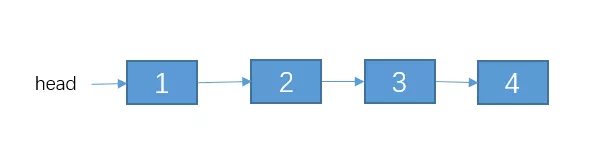Node reverseList(Node head){
}
// 我们先把递归的结果保存起来，先不返回，因为我们还不清楚这样递归是对还是错。，
}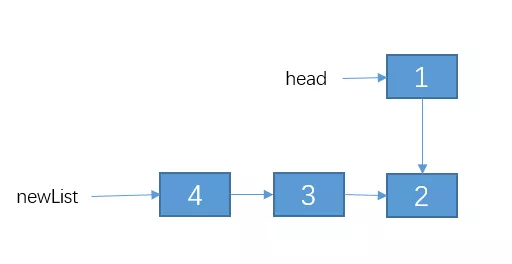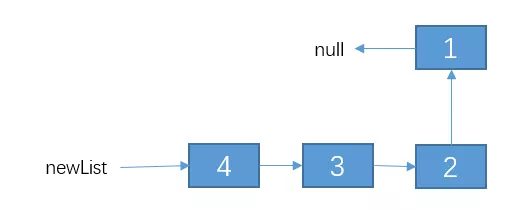//用递归的方法反转链表
// 1.递归结束条件
}
// 递归反转 子链表
// 改变 1，2节点的指向。
// 让 2 的 next 指向 2
// 1 的 next 指向 null.
// 把调整之后的链表返回。
return newList;
}



### 有关递归的一些优化思路

1. 考虑是否重复计算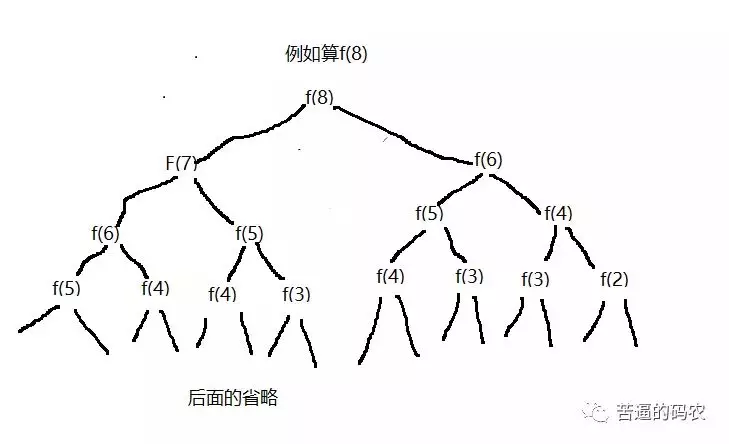// 我们实现假定 arr 数组已经初始化好的了。
int f(int n){
if(n <= 1){
return n;
}
//先判断有没计算过
if(arr[n] != -1){
//计算过，直接返回
return arr[n];
}else{
// 没有计算过，递归计算,并且把结果保存到 arr数组里
arr[n] = f(n-1) + f(n-1);
reutrn arr[n];
}
}


2. 考虑是否可以自底向上

f(1) = 1;

f(2) = 2;

public int f(int n) {
if(n <= 2)
return n;
int f1 = 1;
int f2 = 2;
int sum = 0;

for (int i = 3; i <= n; i++) {
sum = f1 + f2;
f1 = f2;
f2 = sum;
}
return sum;
}


### 最后，献上我备战校招的思维导图 + 提升内功的 PDF 吧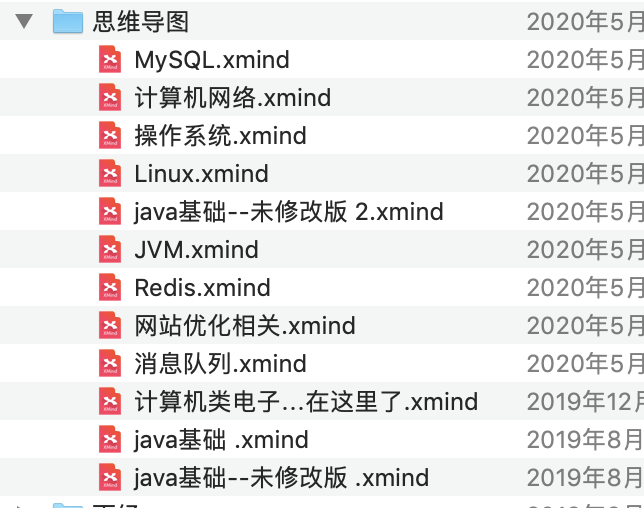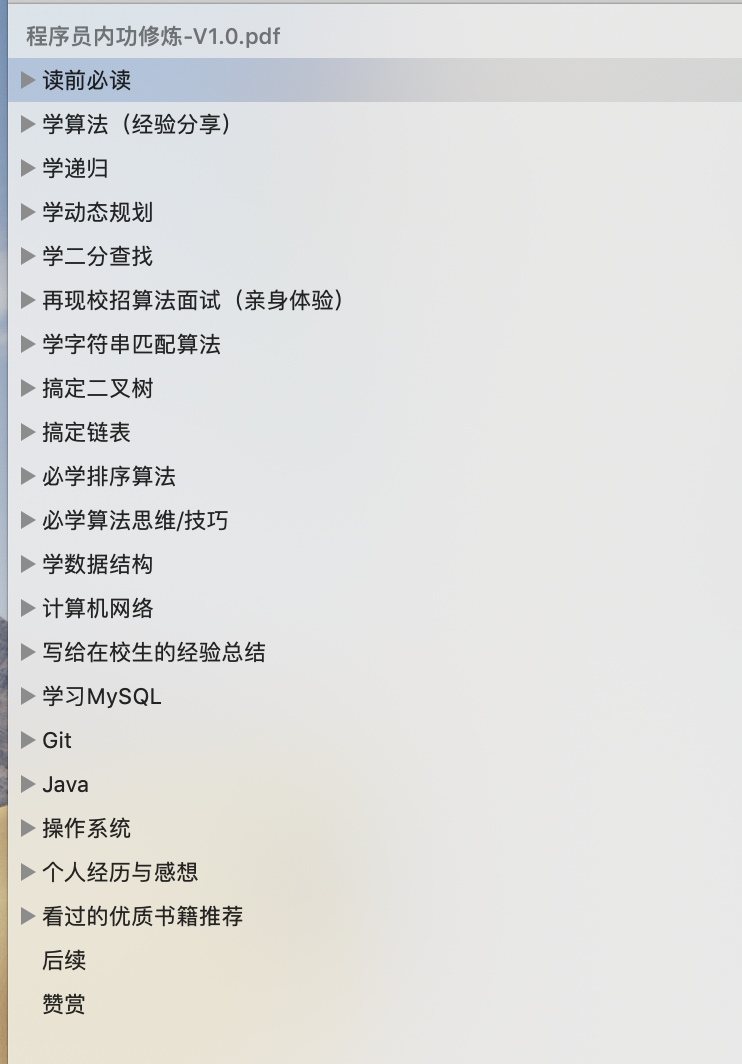11-16105
09-0973
05-13260
11-2064
03-15226
05-08102
03-17378

### “相关推荐”对你有帮助么？

•非常没帮助
•没帮助
•一般
•有帮助
•非常有帮助

©️2022 CSDN 皮肤主题：Age of Ai 设计师：meimeiellie被折叠的  条评论 为什么被折叠?到【灌水乐园】发言¥2 ¥4 ¥6 ¥10 ¥20余额支付 (余额：-- )扫码支付获取中扫码支付点击重新获取扫码支付1.余额是钱包充值的虚拟货币，按照1:1的比例进行支付金额的抵扣。
2.余额无法直接购买下载，可以购买VIP、C币套餐、付费专栏及课程。余额充值